# The mathematical foundation of probability theory

Posted Jun 26, 20208 min read

Compile|VK
Source|Towards Data ScienceAbstraction is to hide irrelevant things and focus only on important details. Although it sometimes looks scary, it is the best tool for managing complexity.

If you let n mathematicians define what math is, you might get 2n different answers. My definition is that it is an abstraction of things until only the core science remains, which provides the final framework for the reasoning of anything.

Have you ever thought about the probability? You must use it to infer data, do statistical analysis, and even build inference algorithms for you through statistical learning. In this article, we will explore probability theory in depth.

#### Pre-knowledge

In order to carry it through, you don't need any advanced mathematics, I will concentrate on explaining everything from the basics. However, it is beneficial if you know the following:

• Sets and set operations, such as union, intersection and difference.
• Limits and some basic calculus.

### Events and metrics

Probability can be thought of as a function heuristically, used to measure the likelihood of an event. But mathematically, it is unclear what events and measures are. Before we can properly discuss probability, we need to lay a solid foundation. So let's start with the event.

#### Event

"What is the probability that I will use this dice to roll odd numbers?"

When we talk about probability, this simple question comes to our mind as an example. In this simple problem, the event is throwing an odd number.

For mathematical modeling, we use sets. The basic set "full set" containing the experimental results is ={1, 2, 3, 4, 5, 6}, and the events are a subset of . Here, throwing odd numbers corresponds to subset A={1, 3, 5}.

Therefore, to define probability, we need a set of base set and its subset , which we call events. However, cannot be just any set of subsets. Three conditions must be met.

• is an event.
• If X is an event, then its complement X is also an event. In other words, an event that did not happen is another event.
• The combination of events must also be an event. In other words, the combination of events and other events is also an event.

If these conditions are met, is called -algebra. Use appropriate mathematical terms: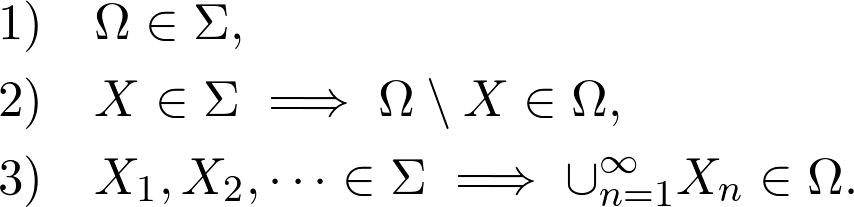In our case, we have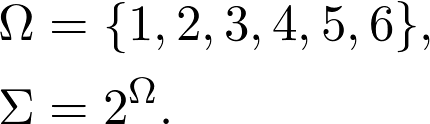When is a set of real numbers, a more interesting situation arises. We will see later that if all subsets of real numbers are treated as events, then very strange things will happen.

#### Describe -algebra

These event spaces defined by -algebra are difficult to describe. We can immediately see that in order to have a meaningful event space on a non-trivial basis set , we should have an infinite number of events.

For example, we fire bullets on a board and want to calculate the probability of hitting a certain area. In these cases, it is sufficient to specify some subsets and take the smallest -algebra that contains these subsets.

Suppose we are shooting a rectangular plate. If we say that our event space is the smallest -algebra of all rectangular subsets containing plates, then we

1. There is a very simple description of -algebra,
2. There will be various shapes because -algebra is closed under union.

Many sets can be described as an infinite union of rectangles, as shown below.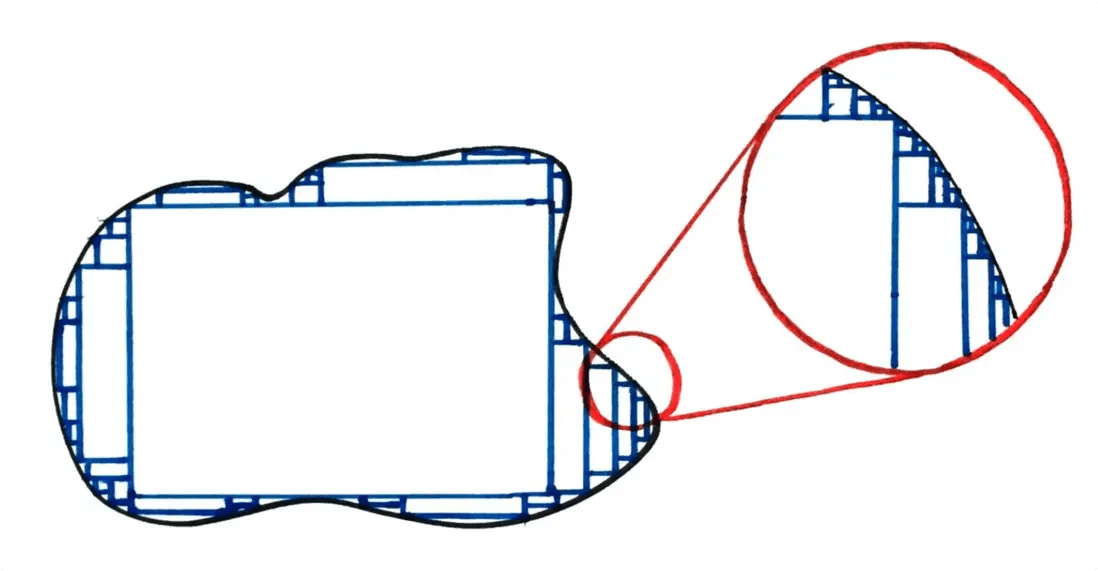We call the rectangular set in the board a generating set, and the smallest -algebra is the generating -algebra.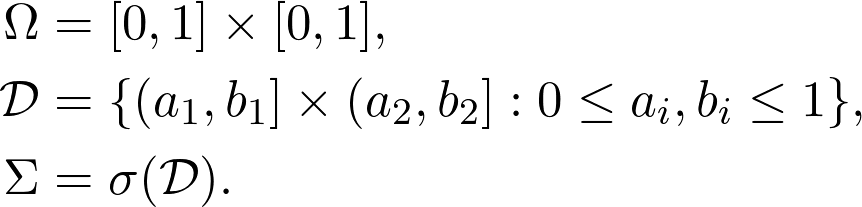You can think of this generation process as getting all the elements of the generation set, and getting the union and complement sets in all possible ways.

Now that we have a mathematical framework for handling events, we should turn our attention to measurement.

#### Measurement

Although it is clear to measure something intuitively, it is very difficult to formalize. A metric is basically a function that maps a set to a number. To give a simple example, measuring the volume of a three-dimensional object seems simple, but even here, we have serious problems. Can you think of an object that you cannot measure the area?

Maybe you can t do it right away, but definitely not. It can be seen that if each subset of space has a well-defined volume, then a sphere of unit volume can be taken, divided into several blocks, and two spheres of unit volume can be put together.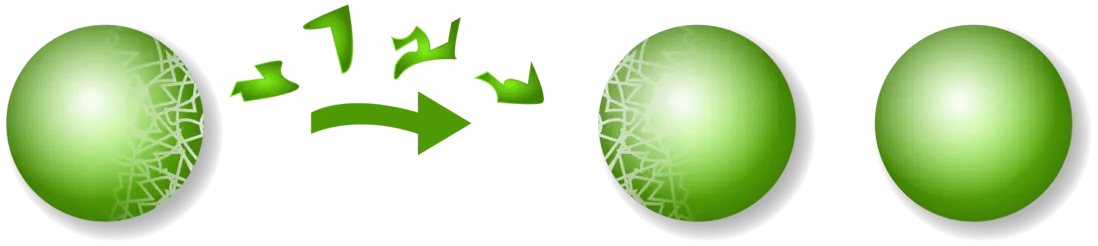This is the so-called Banach-Tarski paradox. Since you cannot really do this, you cannot measure the volume of each subset in space.

But in this case, what measures are there? In fact, we only have three conditions:

1. A metric value should always be positive;
2. The metric value of the empty set should be zero;
3. If you add up the measures of disjoint sets, you get the measure of their union.

In order to define them correctly, we need the base set and -algebra of the subset. function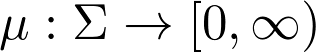Is a measure ifAttribute 3. It is called -additivity. If we only have a limited set, we will simply call it additive of the metric.

This definition is just an abstraction of volumetric measurement. This may seem strange, but these three attributes are the most important. Everything else came from them. For example, we have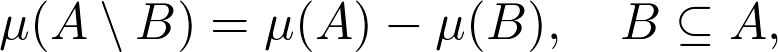This is because AB and B are disjoint and their union is A.Another important property is the continuity of measurement. That is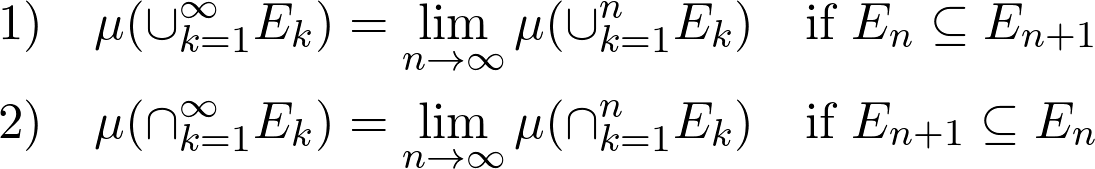This attribute is similar to the definition of continuity of real-valued functions, so naming is not accidental.

#### Describe metrics

As we saw in -algebra, you only need to give a generating set, not a complete -algebra. This is very useful for our measures. Although the metric is defined on -algebra, it is sufficient to define the metric on the generated subset, because -additivity determines the measure of each element in -algebra.

### Definition of Probability

Everything is now set to mathematically define probability.

The probability space is defined by tuples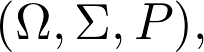Where is the base set, is the -algebra of its subset, and P is such a metric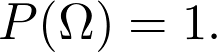Therefore, probability is closely related to area and volume. Area, volume and probability are all measured in their respective spaces. However, this is a fairly abstract concept, so let us give a few examples.

#### toss a coin

The simplest probability space is described by a coin flip event. Suppose we code the positive side with 0 and the negative side with 1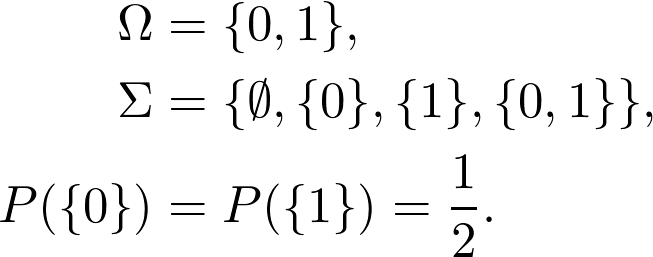Due to the nature of -algebras and measures, you only need to define the probabilities of event {0}(head) and event {1}(tail), which completely determines the probability measure.

#### random number

A more interesting example is random number generation. If you are familiar with Python, you may have used a random function, which gives you a random number between 0 and 1. Although this may seem mysterious, it is fairly simple to describe it in probability space.Note again that this is enough to give the probability of each element of the generated set. For example, we have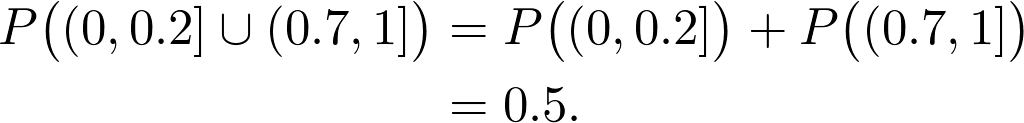To see a more complicated example, what is P({0.5})? How do we calculate the probability of choosing 0.5?(Or any other number between 0 and 1) For this, we need to rely on the properties of the metric. We have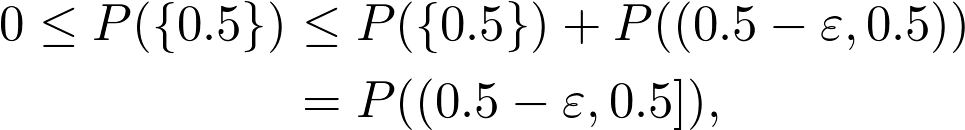Among them, this applies to all >0. Here, we use the additivity of the probability measure. Therefore, this means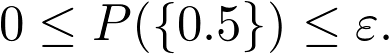Similarly, because it applies to all >0. This means that the probability is less than any positive real number, so it must be zero.

There is a similar argument for any 0 x 1. It may be surprising to see that the probability of choosing a particular number is zero. Therefore, after generating a random number and observing the result, it is necessary to know that the probability of its occurrence is exactly zero. However, there is a conclusion before you.

Zero probability events are possible.

### Distribution and density

We have come a long way. However, from a practical point of view, it is not very convenient to use measures and -algebras. Fortunately, this is not the only way to deal with probability.

For simplicity, assume that our base set is a real number set. Specifically, we have a probability space(, , P), whereP is any measure of probability in this space. We have seen before that the probability of events(a, b) determines the probability of other events in the event space. However, we can further compress this information. In fact, the function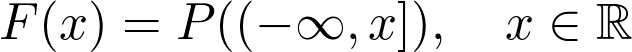Contains all the information we must know about the probability measure. Think about it:we have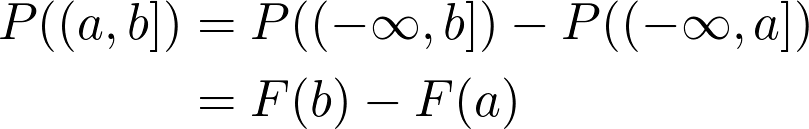For all a and b, this is called the distribution function of P. For all probability measures, the distribution function satisfies the following properties: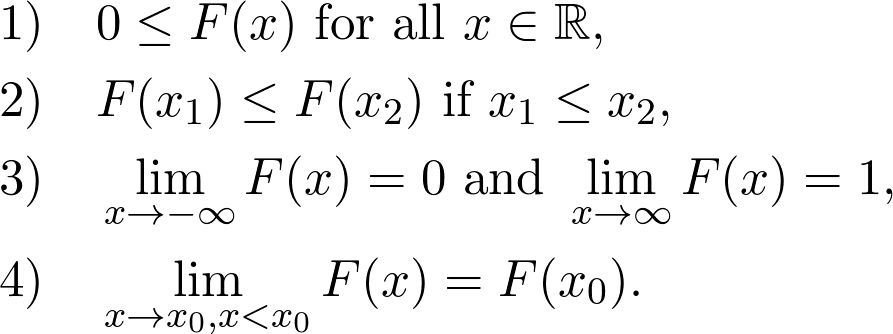(The fourth is called left continuity. Don t emphasize that if you are not familiar with the definition of continuity, you don t need it now.)

Again, if this is too abstract, let us consider an example. For the previous random number generation example, we have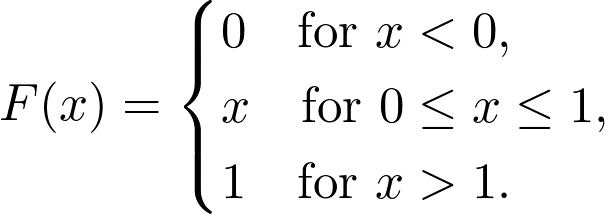This is called the uniform distribution on [0,1].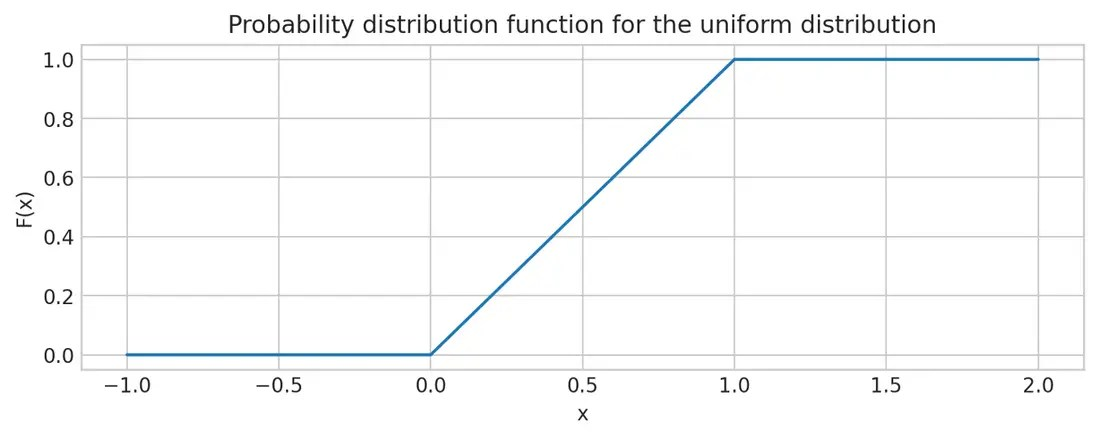In short, if you give me a measure of probability, I will give you a distribution function that describes the measure of probability.

However, this is not the best choice about the distribution function. From a mathematical point of view, if you give a function that satisfies the properties of 1 4 above, I can also use it to construct a probability measure. In addition, if the two distribution functions are equal everywhere, their corresponding probability measures are also the same.

Therefore, from a mathematical point of view, the distribution function and the probability measure are the same in some cases. This is very useful to us.

#### Density function

As we have seen, the distribution function takes all information from the probability measure and compresses it. This is a good tool, but sometimes inconvenient. For example, when we only have a distribution function, it is difficult to calculate the expected value.(If you don t know the expected value, don t worry, we won t use it now.)

In many practical applications, we use density functions to describe probability measures. functionIs the density function of the probability measure P, ifApplies to all E in -algebra . That is, heuristically, the probability of a given set is determined by the area under the f(x) curve. This definition may seem simple, but many details are hidden here, and I don t want to discuss it in detail.

You may be familiar with the famous Newton-Leibniz law in calculus. Here, that is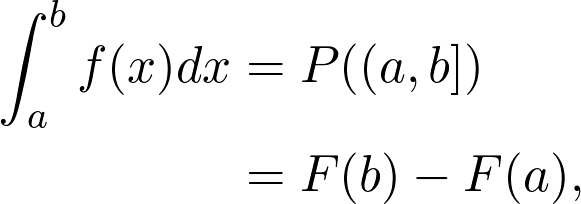This basically means that if the distribution function is differentiable, its derivative is the density function.

There is a certain probability distribution, of which only the density function is a known closed form.(Having a closed form means that it can be represented by a limited number of standard operations and elementary functions.) One of the most famous distributions is this:the Gaussian distribution. Its definition is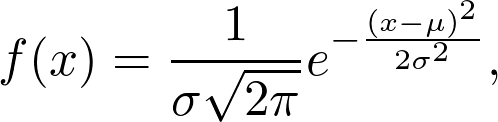Where and are parameters.

Density function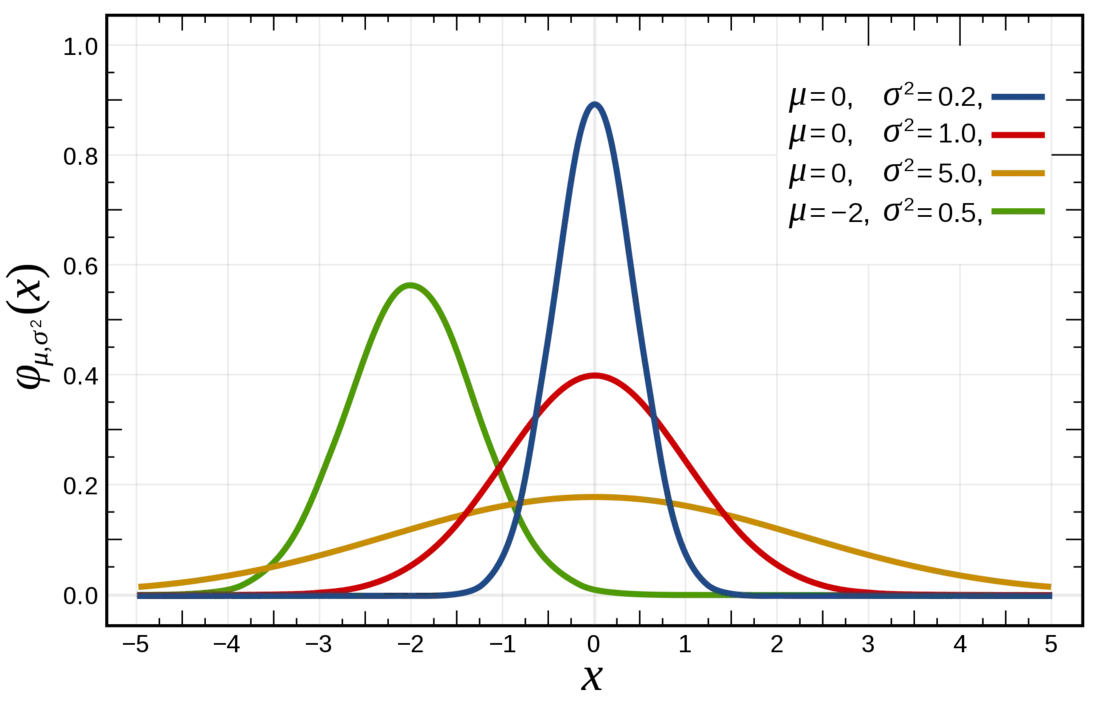Distribution functionNo matter how surprising it may seem, we cannot represent the Gaussian distribution function in a closed form. It is not that mathematicians have not figured it out, but it proves that this is impossible.(Trust me, it is sometimes extremely difficult to prove things that cannot be done mathematically.)

### end

So far, all we have seen is the tip of the iceberg.(Think about it, this can be said at the end of every discussion about mathematics) Here, we only define what is probability in a mathematical(semi)accurate way.

Really interesting things, such as machine learning, are still in front of us.